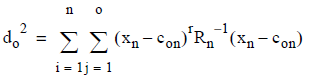# 5.4.5 - Expectation Step - Teradata Warehouse Miner

## Teradata Warehouse Miner User Guide - Volume 3Analytic Functions

Product
Release Number
5.4.5
Published
February 2018
Language
English (United States)
Last Update
2018-05-04
dita:mapPath
yuy1504291362546.ditamap
dita:ditavalPath
ft:empty

Means, variances and frequencies of rows assigned by cluster are first calculated. A covariance inverse matrix is then constructed using these variances, with all non-diagonals assumed to be zero. This simplification is tantamount to the assumption that the variables are independent. Performance is improved thereby, allowing the number of calculations to be proportional to the number of variables, rather than its square. Row distances to the mean of each cluster are calculated using a Mahalanobis Distance (MD) metric:where the following is true:
• m is the number of rows
• n is the number of variables
• o is the number of clusters
• d is dimensioned n by o and is the Mahalanobis Distance from a row to a cluster
• x is dimensioned m by n and is the data
• c is dimensioned 1 by n and are the cluster centroids
• R is dimensioned n by n and is the cluster variance/covariance matrix

Mahalanobis Distance is a rescaled unitless data form used to identify outlying data points. Independent variables may be thought of as defining a multidimensional space in which each observation can be plotted. Means (“centroids”) for each independent variable may also be plotted. Mahalanobis distance is the distance of each observation from its centroid, defined by variables that may be dependent. In the special case where variables are independent or uncorrelated, it is equivalent to the simple Euclidean distance. In the default GM model, separate covariance matrices are maintained, conforming to the specifications of a pure maximum likelihood rule model.

The EM algorithm works by performing the expectation and maximization steps iteratively until the log-likelihood value converges (i.e., changes less than a default or specified epsilon value), or until a maximum specified number of iterations has been performed. The log-likelihood value is the sum over all rows of the natural log of the probabilities associated with each cluster assignment. Although the EM algorithm is guaranteed to converge, it is possible it may converge slowly for comparatively random data, or it may converge to a local maximum rather than a global one.